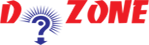﻿ Python Program to Find the Factorial of a Number## Python Program To Find the Factorial Number

Here in this tutorial, we will tell you how to find the fictorial of a number. Through this Python example, you will get complete knowledge of this Python program.

The factorial of a number is basically the multiplication of all the integers starting from 1 to that number.

``````
For example:
The factorial of 5 (5!) is 5*4*3*2*1 = 120
```
```

Note: The factorial of negative numbers are not defined and the factorial of 0 (0!) is 1.

``````
Python Code
# Python program to find the factorial of a number provided by the user.

# change the value for a different result
num = 8

# uncomment to take input from the user
#num = int(input("Enter a number: "))

factorial = 1

# check if the number is negative, positive or zero
if num < 0:
print("Sorry, factorial does not exist for negative numbers")
elif num == 0:
print("The factorial of 0 is 1")
else:
for i in range(1,num + 1):
factorial = factorial*i
print("The factorial of",num,"is",factorial)
Output
The factorial of 8 is 40320
```
```

Note: If you want to test this program, change the values and run it. Also, you can try negative numbers in this program.

## Other Python Programs

PythonTraining, 258-FF , Katewa Nagar, New Sanganer Rd, Jaipur, 302019